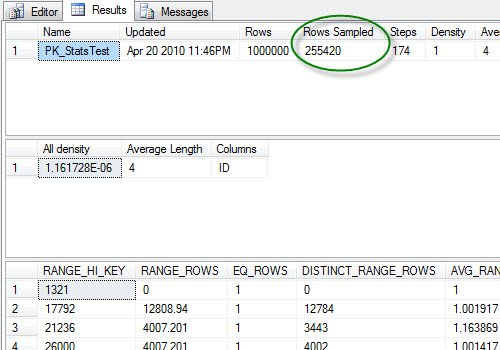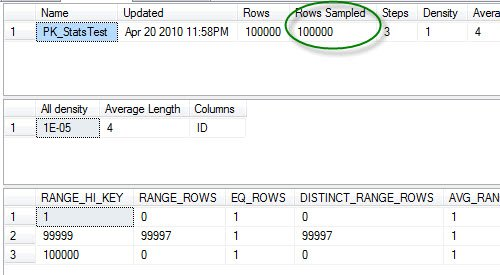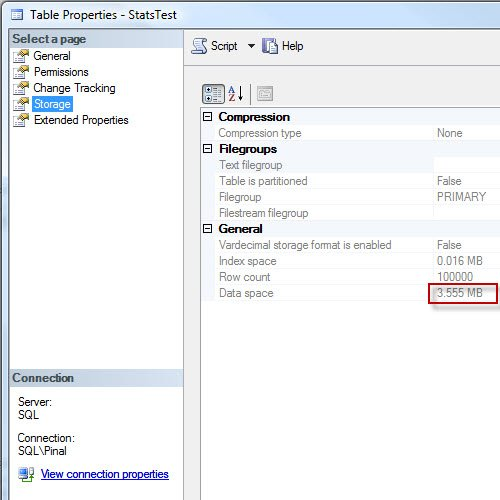# SQL SERVER – Update Statistics are Sampled By Default

After reading my earlier post SQL SERVER – Create Primary Key with Specific Name when Creating Table on Statistics, I have received another question by a blog reader. The question is as follows:

Question: Are the statistics sampled by default?
Answer: Yes. The sampling rate can be specified by the user and it can be anywhere between a very low value to 100%.

Let us do a small experiment to verify if the auto update on statistics is left on. Also, let’s examine a very large table that is created and statistics by default- whether the statistics are sampled or not.

```USE [AdventureWorks] GO -- Create Table CREATE TABLE [dbo].[StatsTest]( [ID] [int] IDENTITY(1,1) NOT NULL, [FirstName] [varchar](100) NULL, [LastName] [varchar](100) NULL, [City] [varchar](100) NULL, CONSTRAINT [PK_StatsTest] PRIMARY KEY CLUSTERED ([ID] ASC) ) ON [PRIMARY] GO -- Insert 1 Million Rows INSERT INTO [dbo].[StatsTest] (FirstName,LastName,City) SELECT TOP 1000000 'Bob', CASE WHEN ROW_NUMBER() OVER (ORDER BY a.name)%2 = 1 THEN 'Smith' ELSE 'Brown' END, CASE WHEN ROW_NUMBER() OVER (ORDER BY a.name)%10 = 1 THEN 'New York' WHEN ROW_NUMBER() OVER (ORDER BY a.name)%10 = 5 THEN 'San Marino' WHEN ROW_NUMBER() OVER (ORDER BY a.name)%10 = 3 THEN 'Los Angeles' ELSE 'Houston' END FROM sys.all_objects a CROSS JOIN sys.all_objects b GO -- Update the statistics UPDATE STATISTICS [dbo].[StatsTest] GO -- Shows the statistics DBCC SHOW_STATISTICS ("StatsTest",PK_StatsTest) GO -- Clean up DROP TABLE [dbo].[StatsTest] GO ```

Now let us observe the result of the `DBCC SHOW_STATISTICS`.The result shows that Resultset is for sure sampling for a large dataset. The percentage of sampling is based on data distribution as well as the kind of data in the table. Before dropping the table, let us check first the size of the table. The size of the table is 35 MB.Now, let us run the above code with lesser number of the rows.

```USE [AdventureWorks] GO -- Create Table CREATE TABLE [dbo].[StatsTest]( [ID] [int] IDENTITY(1,1) NOT NULL, [FirstName] [varchar](100) NULL, [LastName] [varchar](100) NULL, [City] [varchar](100) NULL, CONSTRAINT [PK_StatsTest] PRIMARY KEY CLUSTERED ([ID] ASC) ) ON [PRIMARY] GO -- Insert 1 Hundred Thousand Rows INSERT INTO [dbo].[StatsTest] (FirstName,LastName,City) SELECT TOP 100000 'Bob', CASE WHEN ROW_NUMBER() OVER (ORDER BY a.name)%2 = 1 THEN 'Smith' ELSE 'Brown' END, CASE WHEN ROW_NUMBER() OVER (ORDER BY a.name)%10 = 1 THEN 'New York' WHEN ROW_NUMBER() OVER (ORDER BY a.name)%10 = 5 THEN 'San Marino' WHEN ROW_NUMBER() OVER (ORDER BY a.name)%10 = 3 THEN 'Los Angeles' ELSE 'Houston' END FROM sys.all_objects a CROSS JOIN sys.all_objects b GO -- Update the statistics UPDATE STATISTICS [dbo].[StatsTest] GO -- Shows the statistics DBCC SHOW_STATISTICS ("StatsTest",PK_StatsTest) GO -- Clean up DROP TABLE [dbo].[StatsTest] GO ```

You can see that Rows Sampled is just the same as Rows of the table. In this case, the sample rate is 100%.Before dropping the table, let us also check the size of the table. The size of the table is less than 4 MB.Let us compare the Result set just for a valid reference.

Test 1: Total Rows: 1000000, Rows Sampled: 255420, Size of the Table: 35.516 MB

Test 2: Total Rows: 100000, Rows Sampled: 100000, Size of the Table: 3.555 MB

The reason behind the sample in the Test1 is that the data space is larger than 8 MB, and uses more than 1024 data pages. If the data space is smaller than 8 MB and uses less than 1024 data pages, then the sampling does not happen (or happens 100%). Sampling aids in reducing excessive data scan; however, sometimes it reduces the accuracy of the data as well.

Please note that this is just a sample test and there is no way it can be claimed as a benchmark test. The result can be dissimilar on different machines. There are lots of other information can be included when talking about this subject. I will write detail post covering all the subject very soon. If you something missing please free to add it.

Reference: Pinal Dave (https://blog.sqlauthority.com)

#### Related Posts

•Phani
April 23, 2010 9:09 am

Good stuff. I think based on the data growth rate in a table it would be adviced to build statistics with 100% sampling to make sure that the statistics are better used. Otherwise, we might end up with a situation where the index is not properly being used because of cardinality maintained in statistics. This is my personal opinion and am open to understand it better if anyone has a different view on the same.

•Paresh Prajapati1
April 23, 2010 2:03 pm

Hi Pinal,

In Test1 , How can we manually change sampling to 100%?

•Duke Lancaster
April 23, 2010 8:05 pm

What is the relationship between statistics and indexes. We have a number of statistics that are duplicates of existing indexes… is this normal or unnecessary?

•Naveena
May 10, 2010 6:04 pm

hi,

i have some error for
DBCC SHOW_STATISTICS (‘tablename’PK_id)
An incorrect number of parameters was given to the DBCC statement.

•Naveena
May 10, 2010 6:04 pm

hi,

Execute this stmt DBCC SHOW_STATISTICS (‘tablename’PK_id). i have some error for

An incorrect number of parameters was given to the DBCC statement.

Regards,
naveena